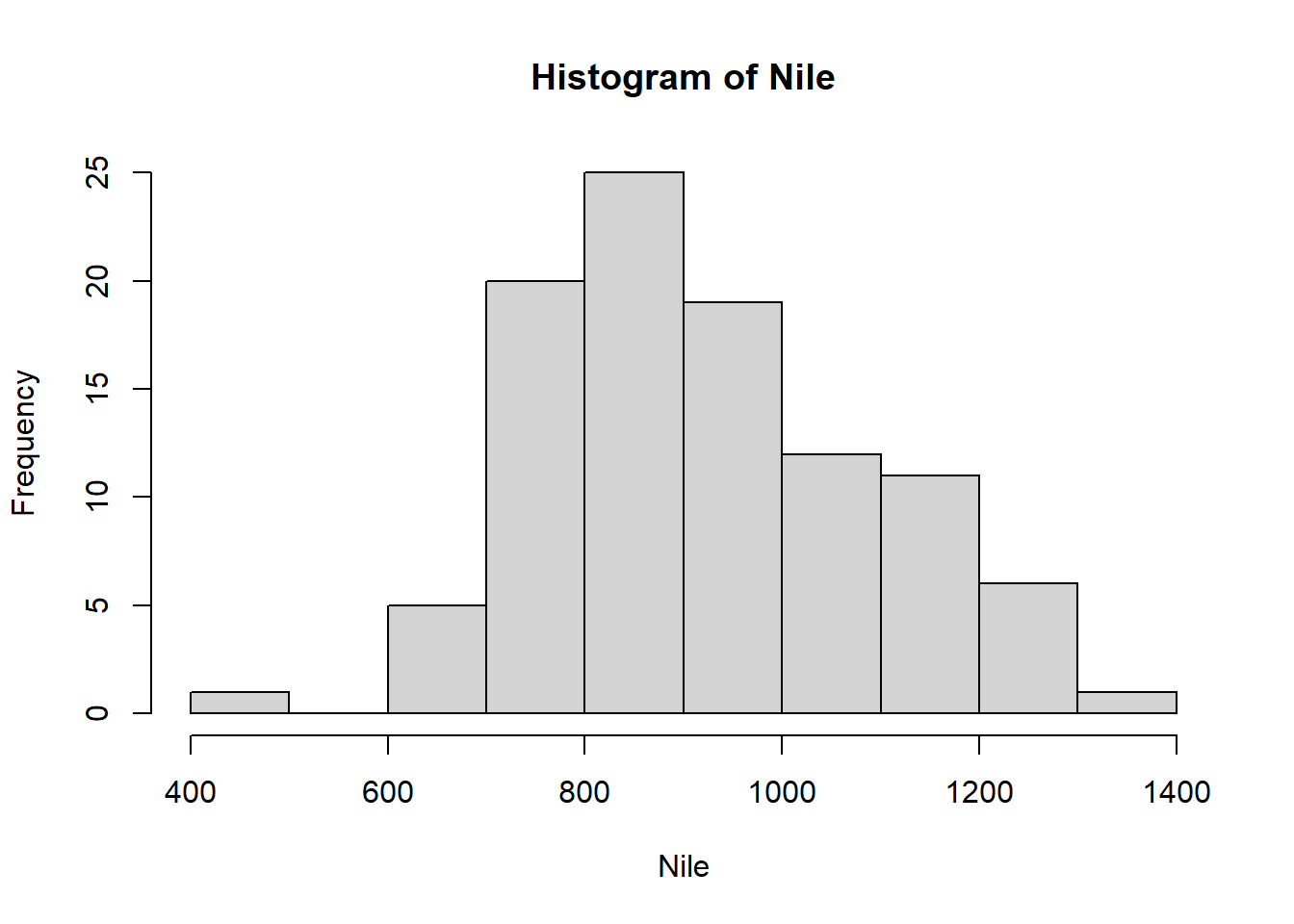## Questions

Exercise: 11-A

Create a dataframe named “df” which is equal to the first three columns and the first five rows of the “mtcars” dataset. Next, rename the “mpg” column to “miles_per_gallon”.

After printing the resulting dataframe to the console you should have the following results:

``````#                   miles_per_gallon cyl disp
# Mazda RX4                     21.0   6  160
# Mazda RX4 Wag                 21.0   6  160
# Datsun 710                    22.8   4  108
# Hornet 4 Drive                21.4   6  258
# Hornet Sportabout             18.7   8  360``````
Exercise: 12-A

You are given the following dataframe:

``````var_1 <- c(3, 4, 2, 9, NA, 2, 7)
var_2 <- c(8, NA, 6, 4, 8, 5, 5)
df <- data.frame(var_1 = var_1, var_2 = var_2)
print(df)
#   var_1 var_2
# 1     3     8
# 2     4    NA
# 3     2     6
# 4     9     4
# 5    NA     8
# 6     2     5
# 7     7     5``````

Create a new dataframe called “cleaned_df” which is equal to “df” except with both rows which contain “NA” values removed.

The final output of “cleaned_df” should look like this:

``````#   var_1 var_2
# 1     3     8
# 3     2     6
# 4     9     4
# 6     2     5
# 7     7     5``````
Exercise: 12-B

Take the original “df” dataframe from exercise 12-A and apply a constant value of “5” to each “NA” value. Store this new dataframe in a variable named “constant_value”.

Your final output after printing “constant_value” to the console should look like this:

``````print(constant_value)
#   var_1 var_2
# 1     3     8
# 2     4     5
# 3     2     6
# 4     9     4
# 5     5     8
# 6     2     5
# 7     7     5``````
Exercise: 12-C

Take the same “df” dataframe from exercises 12-A and 12-B and apply an average value of each column to “NA” values in each respective column. Store this new dataframe in a variable named “mean_value”.

Your final output after printing “mean_value” to the console should look like this:

``````print(mean_value)
#   var_1 var_2
# 1   3.0     8
# 2   4.0     6
# 3   2.0     6
# 4   9.0     4
# 5   4.5     8
# 6   2.0     5
# 7   7.0     5``````
Exercise: 13-A

Use the “Nile” dataset to create a histogram to view the distribution of it’s data.

Exercise: 14-A

Take the dataframe created in exercise 11-A and drop any row where the “disp” column is equal to “160”.

You should receive the following results when you print the resulting dataframe to the console.

``````#                   miles_per_gallon cyl disp
# Datsun 710                    22.8   4  108
# Hornet 4 Drive                21.4   6  258
# Hornet Sportabout             18.7   8  360``````

This task could be accomplished in the following way:

``library(dplyr)``
``````
Attaching package: 'dplyr'``````
``````The following objects are masked from 'package:stats':

filter, lag``````
``````The following objects are masked from 'package:base':

intersect, setdiff, setequal, union``````
``````df <- mtcars[1:5, 1:3]
df <- rename(df, "miles_per_gallon" = "mpg")
print(df)``````
``````                  miles_per_gallon cyl disp
Mazda RX4                     21.0   6  160
Mazda RX4 Wag                 21.0   6  160
Datsun 710                    22.8   4  108
Hornet 4 Drive                21.4   6  258

This task could be accomplished in the following way:

``````var_1 <- c(3, 4, 2, 9, NA, 2, 7)
var_2 <- c(8, NA, 6, 4, 8, 5, 5)
df <- data.frame(var_1 = var_1, var_2 = var_2)
cleaned_df <- na.omit(df)
print(cleaned_df)``````
``````  var_1 var_2
1     3     8
3     2     6
4     9     4
6     2     5
7     7     5``````

There are several ways this task could be accomplished; however, the following example demonstrates one way to do it.

``````var_1 <- c(3, 4, 2, 9, NA, 2, 7)
var_2 <- c(8, NA, 6, 4, 8, 5, 5)
df <- data.frame(var_1 = var_1, var_2 = var_2)

constant_value <- df
constant_value[is.na(constant_value)] <- 5
print(constant_value)``````
``````  var_1 var_2
1     3     8
2     4     5
3     2     6
4     9     4
5     5     8
6     2     5
7     7     5``````

There are several ways this task could be accomplished; however, the following example demonstrates one way to do it.

``````var_1 <- c(3, 4, 2, 9, NA, 2, 7)
var_2 <- c(8, NA, 6, 4, 8, 5, 5)
df <- data.frame(var_1 = var_1, var_2 = var_2)

mean_1 <- mean(df\$var_1[!is.na(df\$var_1)])
mean_2 <- mean(df\$var_2[!is.na(df\$var_2)])

mean_value <- df
mean_value\$var_1[is.na(mean_value\$var_1)] <- mean_1
mean_value\$var_2[is.na(mean_value\$var_2)] <- mean_2
print(mean_value)``````
``````  var_1 var_2
1   3.0     8
2   4.0     6
3   2.0     6
4   9.0     4
5   4.5     8
6   2.0     5
7   7.0     5``````
``hist(Nile)````````library(dplyr)
``````                  miles_per_gallon cyl disp